``````library(ggcyto)

## 1: suppoort `3` types of plot constructor

• represent different levels of complexity and flexibility
• meet the needs of various plot applications
• suitable for users at different levels of coding skills.

### low level: `ggplot`

The overloaded `fority` methods empower `ggplot` to work with all the major Cytometry data structures right away, which allows users to do all kinds of highly customized and versitled plots.

#### `GatingSet`

``````gs <- load_gs(list.files(dataDir, pattern = "gs_manual",full = TRUE))
attr(gs, "subset") <- "CD3+"
ggplot(gs, aes(x = `<B710-A>`, y = `<R780-A>`)) + geom_hex(bins = 128) + scale_fill_gradientn(colours = gray.colors(9))``````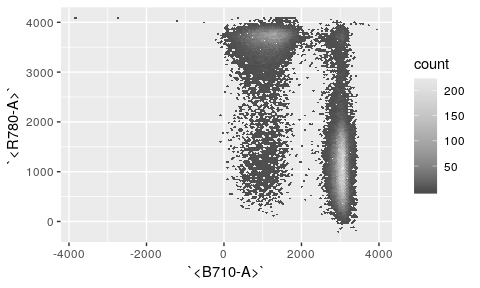#### `flowSet/ncdfFlowSet/flowFrame`

``````fs <- getData(gs, "CD3+")
ggplot(fs, aes(x = `<B710-A>`)) + geom_density(fill = "blue", alpha= 0.5)``````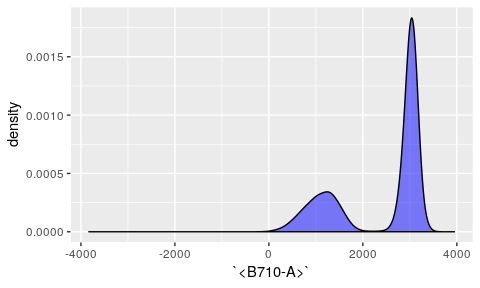#### `gates`

``````gates <- filterList(getGate(gs, "CD8"))
ggplot(gs, aes(x = `<B710-A>`, y = `<R780-A>`)) + geom_hex(bins = 128) + geom_polygon(data = gates, fill = "transparent", col = "purple")``````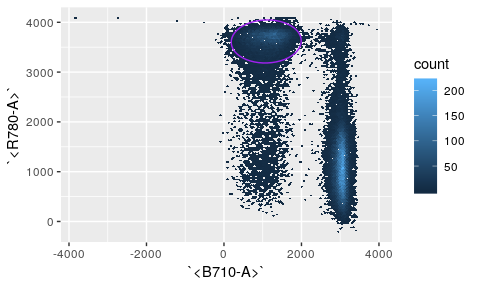### medium level: `ggcyto`

`ggcyto` constructor along with overloaded `+` operator encapsulate lots of details that might be tedious and intimidating for many users.

``ggcyto(gs, aes(x = CD4, y = CD8)) + geom_hex(bins = 128) + geom_gate("CD8")``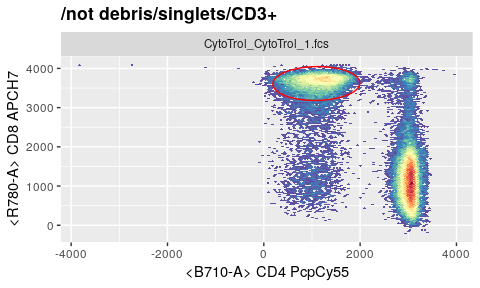It simplies the plotting by: * add a default scale_fill_gradientn for you * fuzzy-matching in `aes` by either detector or fluorochromes names * determine the `parent` popoulation automatically * exact and plot the gate object by simply referring to the `child` population name

### top level: `autoplot`

Inheriting the spirit from ggplot’s `Quick plot`, it further simply the plotting job by hiding more details from users and taking more assumptions for the plot.

• when plotting `flowSet`, it determines `geom` type automatically by the number of `dim` supplied
• for `GatingSet`, it further skip the need of `dim` by guessing it from the `children` gate
``````#1d
autoplot(fs, "CD4")``````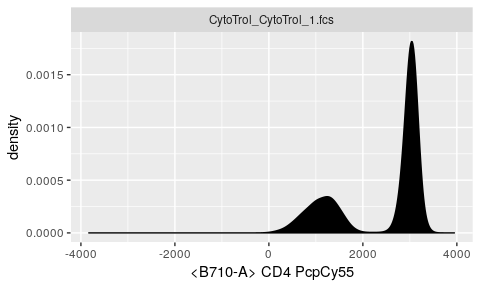``````#2d
autoplot(fs, "CD4", "CD8", bins = 64)``````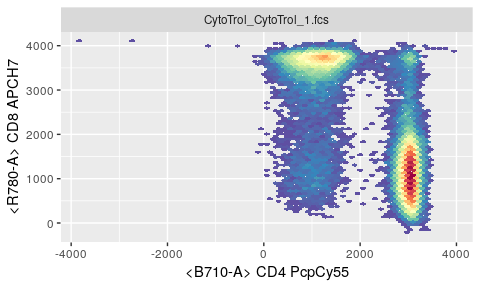``autoplot(gs, c("CD4", "CD8"), bins = 64)``